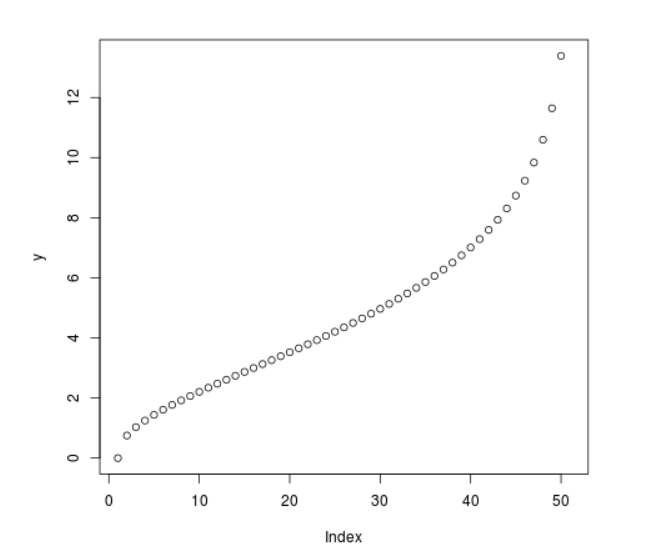Open in App
Not now

# Compute value of Quantile Chi Square Density in R Programming – qchisq() Function

• Last Updated : 25 Jun, 2020

`qchisq()` function in R Language is used to compute value of chi square quantile function. It creates a quantile function plot for chi square distribution.

Syntax: qchisq(vec, df)

Parameters:
vec: Vector of x-values
df: Degree of Freedom

Example 1:

 `# R program to compute ``# Quantile Chi Square density`` ` `# Create a vector of x-values``x <``-` `seq(``0``, ``1``, by ``=` `0.1``)`` ` `# Calling qchisq() Function``y <``-` `qchisq(x, df ``=` `5``)``y`

Output:

```  0.000000 1.610308 2.342534 2.999908 3.655500 4.351460 5.131867 6.064430
 7.289276 9.236357      Inf
```

Example 2:

 `# R program to compute ``# Quantile Chi Square density`` ` `# Create a vector of x-values``x <``-` `seq(``0``, ``1``, by ``=` `0.02``)`` ` `# Calling qchisq() Function``y <``-` `qchisq(x, df ``=` `5``)`` ` `# Plot a graph``plot(y)`

Output:My Personal Notes arrow_drop_up